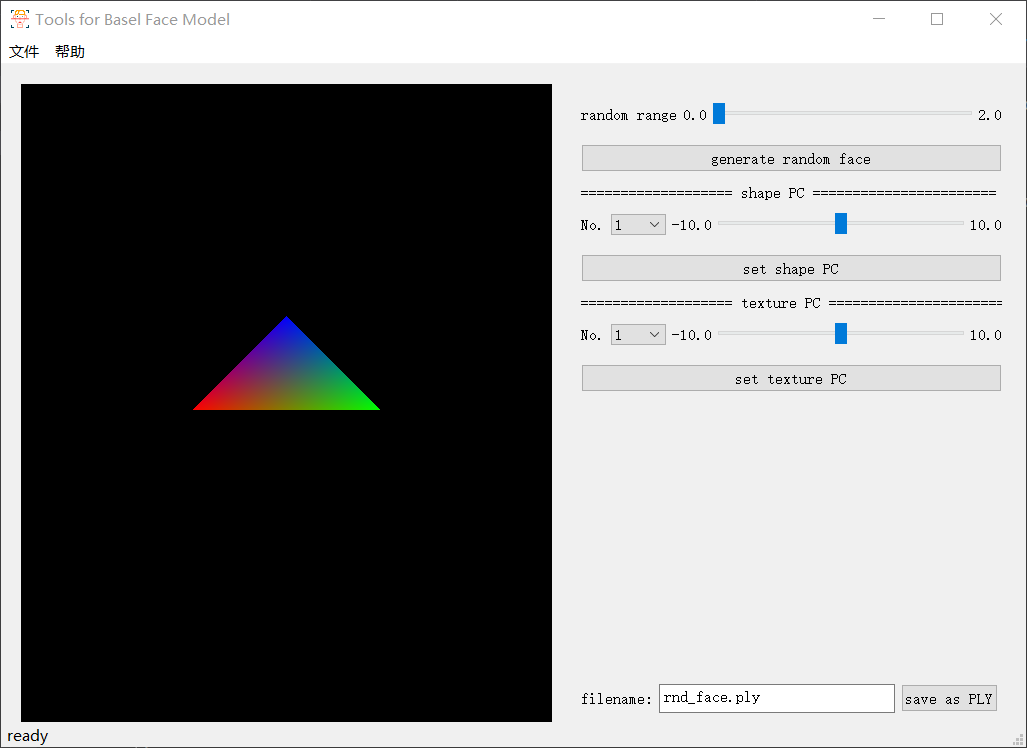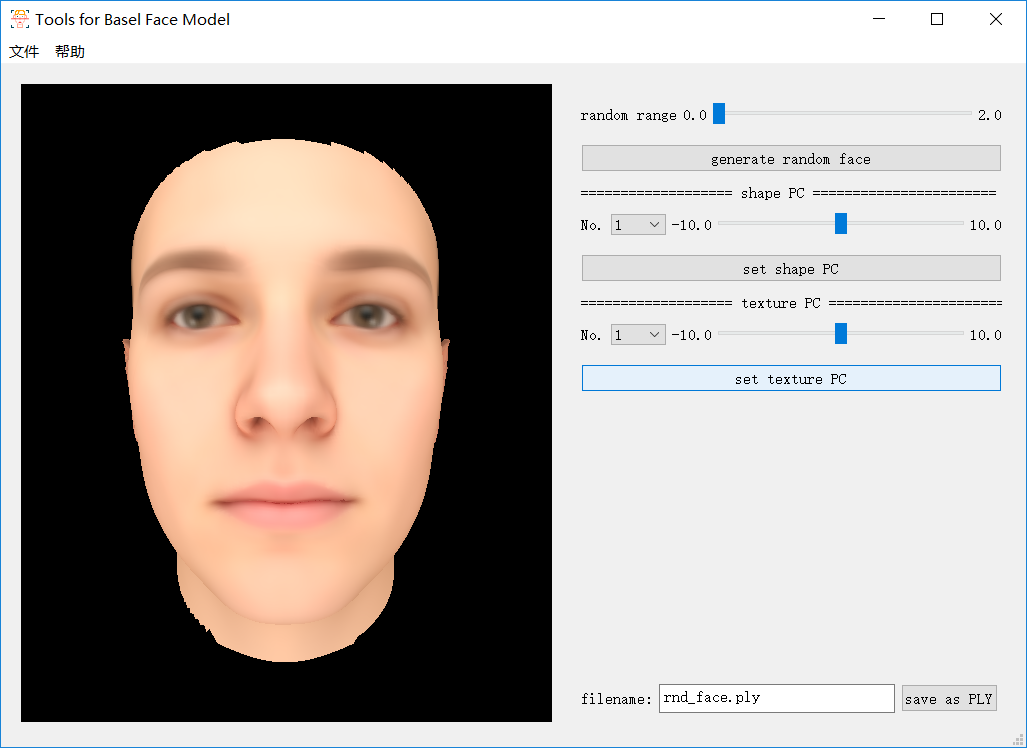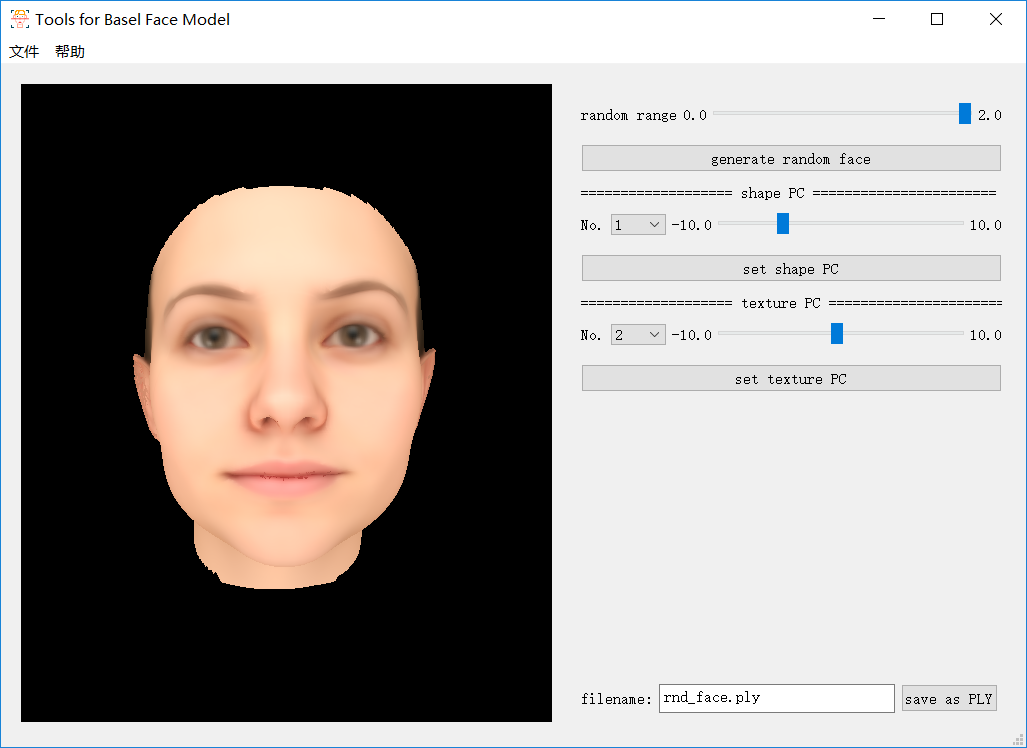# BFM模型介绍及可视化实现（C++）

## BFM模型基本介绍

Basel Face Model是一个开源的人脸数据库，其基本原理是3DMM，因此其便是在PCA的基础上进行存储的。

### 数据内容（以2009版本为例）

#### 文件内容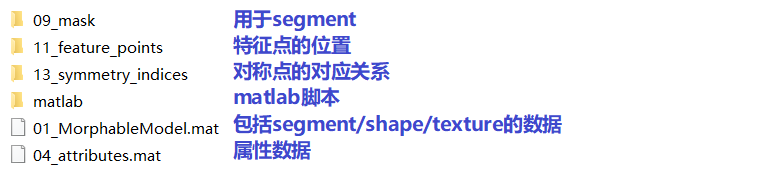#### 01_MorphableModel.mat（数据主体）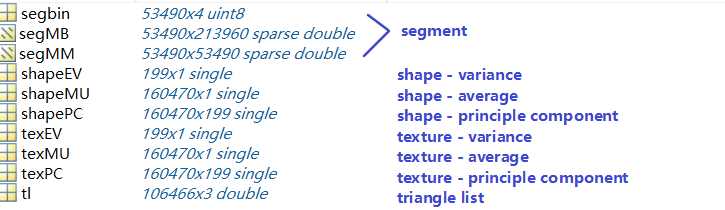BFM模型由53490个顶点构成，其shape/texture的数据长度为160470（53490*3），因为其排列方式如下：

``shape: x_1, y_1, z_1, x_2, y_2, z_2, ..., x_{53490}, y_{53490}, z_{53490}``
``texture: r_1, g_1, b_1, r_2, g_2, b_2, ..., r_{53490}, g_{53490}, b_{53490}``

#### .h5文件与.mat文件对应关系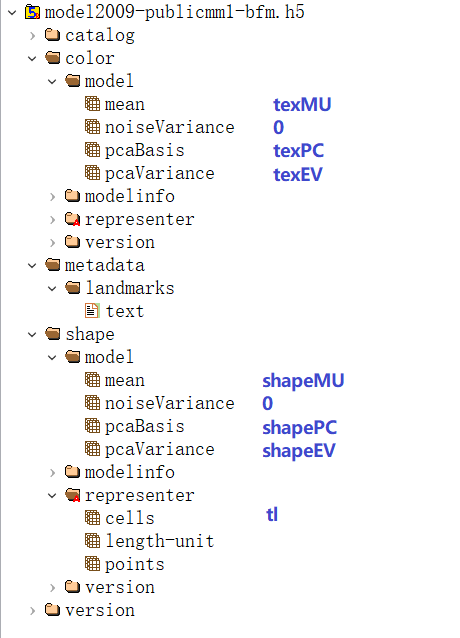[注] .h5文件中的tl数量与.mat数量不同，主成分方差的值也不同，且shape的值是.mat中shape值的0.001倍（见`/shape/representer/length-unit`）。

### 2009与2017版本区别

2009年版本数据集：

• 提供数据格式：mat（`01_MorphableModel.mat`）和h5（`model2009-publicmm1-bfm.h5`）；
• 提供一系列Matlab脚本，有生成随机脸等功能；
• 提供多种特征点（`PublicMM1/11_feature_points`）；
• 提供segment的mask（`PublicMM1/09_mask`）；
• 提供对称点的对应关系（`PublicMM1/13_symmetry_indices`）；
• 提供属性（`PublicMM1/04_attributes.mat`
• 不提供表情；

2017年版本数据集：

• 提供数据格式：h5（原版（`model2017-1_bfm_nomouth.h5`）和裁剪过的版本（`model2017-1_face12_nomouth.h5`））；
• 不提供Matlab脚本（本身也无mat格式数据）；
• 提供单种特征点（`metadata/landmarks/text`）；
• 不提供segment、对称点的对应关系和属性；
• 提供表情（`expression`）；

### 基本原理

``obj = average + pc * (coeficient .* pcVariance)``

## C++实现BFM模型可视工具

### 数据读取

#### 读取.h5格式文件

.h5文件无法直接通过文本工具打开，需要下载专门的可视工具，此处我使用了HDFViewHDF5库下载地址

[注] 在Windows的Visual Studio使用shared库需要编译过程定义`H5_BUILT_AS_DYNAMIC_LIB`。（若出现`LINK2001`错误可以添加这个来解决）
[注] static库命名前面以`lib`开头，例如`hdf5.lib`是shared库，`libhdf5.lib`是static库。

``float *shape_mu_raw = new float[N_VERTICE * 3];  H5File file(bfm_h5_path, H5F_ACC_RDONLY);  DataSet shape_mu_data = file.openDataSet("/shape/model/mean");  shape_mu_data.read(shape_mu_raw, PredType::NATIVE_FLOAT);  raw2vector(shape_mu, shape_mu_raw);   // 自行将数组转换成想要存放的格式  shape_mu_data.close();  file.close();``

shape平均值读取后需要再乘以1000才等同于.mat格式的读取。

[注] 因为缺少pdb文件，HDF5中的代码如果报错可能无法进行调试，需要逐行进行错误的排除，常见错误就是类型不匹配或者长度不匹配。

#### 其他读取方式

``function mat2binary(filename, mat, type)      fid=fopen(filename, 'wb');      matrix = mat;                             [m,n]=size(matrix);       for i=1:1:m         for j=1:1:n              fwrite(fid, matrix(i,j), type);         end      end      fclose(fid);  end``

### OpenGL进行显示

``double sint = sin(theta), cost = cos(theta);  for (auto t = tl.begin(); t != tl.end(); t++) {         glBegin(GL_TRIANGLES);         vec3 tmp = *t;         glColor3f(tex[tmp.x].x / 255.0, tex[tmp.x].y / 255.0, tex[tmp.x].z / 255.0);         glVertex3f(shape[tmp.x].x * scale * cost - shape[tmp.x].z * scale * sint, shape[tmp.x].y * scale, shape[tmp.x].x * scale * sint + shape[tmp.x].z * scale * cost);         glColor3f(tex[tmp.y].x / 255.0, tex[tmp.y].y / 255.0, tex[tmp.y].z / 255.0);         glVertex3f(shape[tmp.y].x * scale * cost - shape[tmp.y].z * scale * sint, shape[tmp.y].y * scale, shape[tmp.y].x * scale * sint + shape[tmp.y].z * scale * cost);         glColor3f(tex[tmp.z].x / 255.0, tex[tmp.z].y / 255.0, tex[tmp.z].z / 255.0);         glVertex3f(shape[tmp.z].x * scale * cost - shape[tmp.z].z * scale * sint, shape[tmp.z].y * scale, shape[tmp.z].x * scale * sint + shape[tmp.z].z * scale * cost);         glEnd();  }``

``void OpenGLWidget::resizeGL(int width, int height) {          glViewport(0, 0, width, height);          glMatrixMode(GL_PROJECTION);          glLoadIdentity();          gluPerspective(60.0, (GLfloat)width / (GLfloat)height, 1.0, 600000.0);          glMatrixMode(GL_MODELVIEW);          glLoadIdentity();          gluLookAt(0, 0, 300000.0, 0.0, 0.0, 0.0, 0.0, 1.0, 0.0);  }``

## 结果展示